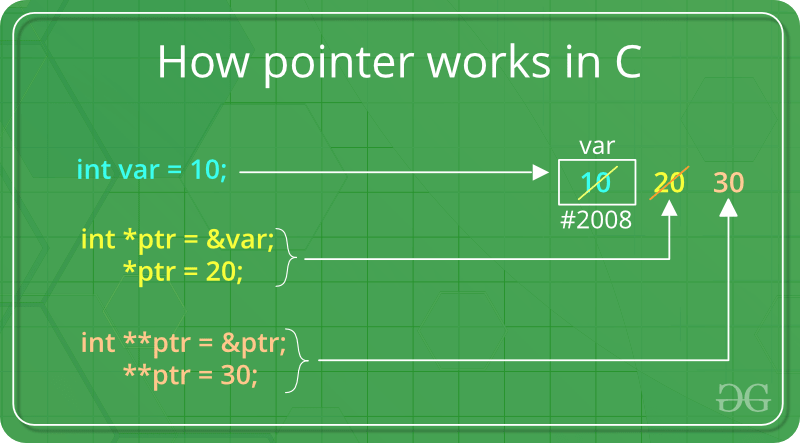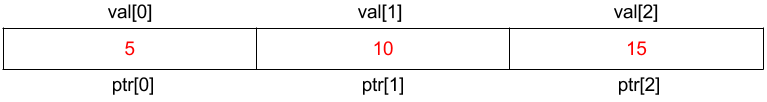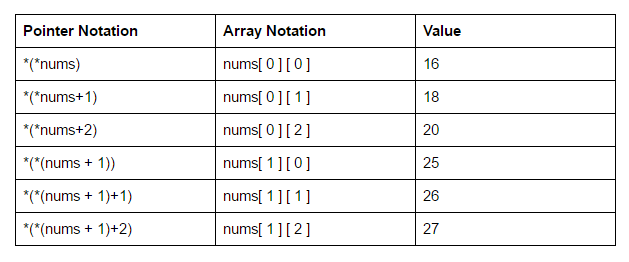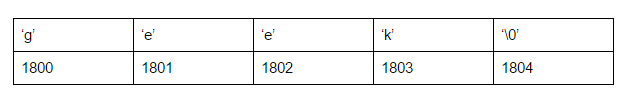# Pointers in C/C++ with Examples

• Difficulty Level : Easy
• Last Updated : 28 Jun, 2021

Pointers are symbolic representation of addresses. They enable programs to simulate call-by-reference as well as to create and manipulate dynamic data structures. It’s general declaration in C/C++ has the format:

Syntax:

```datatype *var_name;
int *ptr;   //ptr can point to an address which holds int data
```How to use a pointer?

• Define a pointer variable
• Assigning the address of a variable to a pointer using unary operator (&) which returns the address of that variable.
• Accessing the value stored in the address using unary operator (*) which returns the value of the variable located at the address specified by its operand.

The reason we associate data type to a pointer is that it knows how many bytes the data is stored in. When we increment a pointer, we increase the pointer by the size of data type to which it points.## C

 `// C++ program to illustrate Pointers in C++``#include `` ` `void` `geeks()``{``    ``int` `var = 20; ``     ` `    ``// declare pointer variable    ``    ``int` `*ptr; ``     ` `    ``// note that data type of ptr and var must be same``    ``ptr = &var;    `` ` `    ``// assign the address of a variable to a pointer``    ``printf``(``"Value at ptr = %p \n"``,ptr);``    ``printf``(``"Value at var = %d \n"``,var);``    ``printf``(``"Value at *ptr = %d \n"``, *ptr);     ``}`` ` `// Driver program``int` `main()``{``    ``geeks();``}`

## C++

 `// C++ program to illustrate Pointers in C++`` ` `#include ``using` `namespace` `std;``void` `geeks()``{``    ``int` `var = 20; ``     ` `        ``//declare pointer variable    ``    ``int` `*ptr; ``     ` `    ``//note that data type of ptr and var must be same``    ``ptr = &var;    `` ` `    ``// assign the address of a variable to a pointer``    ``cout << ``"Value at ptr = "` `<< ptr << ``"\n"``;``    ``cout << ``"Value at var = "` `<< var << ``"\n"``;``    ``cout << ``"Value at *ptr = "` `<< *ptr << ``"\n"``;     ``}``//Driver program``int` `main()``{``    ``geeks();``}`

Output:

```Value at ptr = 0x7ffcb9e9ea4c
Value at var = 20
Value at *ptr = 20
```

References and Pointers

There are 3 ways to pass C++ arguments to a function:

• call-by-value
• call-by-reference with pointer argument
• call-by-reference with reference argument

 `// C++ program to illustrate call-by-methods in C++`` ` `#include ``using` `namespace` `std;``//Pass-by-Value``int` `square1(``int` `n)``{``    ``//Address of n in square1() is not the same as n1 in main()``    ``cout << ``"address of n1 in square1(): "` `<< &n << ``"\n"``;  ``     ` `    ``// clone modified inside the function``    ``n *= n;``    ``return` `n;``}``//Pass-by-Reference with Pointer Arguments``void` `square2(``int` `*n)``{``    ``//Address of n in square2() is the same as n2 in main()``    ``cout << ``"address of n2 in square2(): "` `<< n << ``"\n"``;``     ` `    ``// Explicit de-referencing to get the value pointed-to``    ``*n *= *n;``}``//Pass-by-Reference with Reference Arguments``void` `square3(``int` `&n)``{``    ``//Address of n in square3() is the same as n3 in main()``    ``cout << ``"address of n3 in square3(): "` `<< &n << ``"\n"``;``     ` `    ``// Implicit de-referencing (without '*')``    ``n *= n;``}``void` `geeks()``{``    ``//Call-by-Value``    ``int` `n1=8;``    ``cout << ``"address of n1 in main(): "` `<< &n1 << ``"\n"``;``    ``cout << ``"Square of n1: "` `<< square1(n1) << ``"\n"``;``    ``cout << ``"No change in n1: "` `<< n1 << ``"\n"``;``     ` `    ``//Call-by-Reference with Pointer Arguments``    ``int` `n2=8;``    ``cout << ``"address of n2 in main(): "` `<< &n2 << ``"\n"``;``    ``square2(&n2);``    ``cout << ``"Square of n2: "` `<< n2 << ``"\n"``;``    ``cout << ``"Change reflected in n2: "` `<< n2 << ``"\n"``;``     ` `    ``//Call-by-Reference with Reference Arguments``    ``int` `n3=8;``    ``cout << ``"address of n3 in main(): "` `<< &n3 << ``"\n"``;``    ``square3(n3);``    ``cout << ``"Square of n3: "` `<< n3 << ``"\n"``;``    ``cout << ``"Change reflected in n3: "` `<< n3 << ``"\n"``;``     ` `     ` `}``//Driver program``int` `main()``{``    ``geeks();``}`

Output:

```address of n1 in main(): 0x7ffcdb2b4a44
address of n1 in square1(): 0x7ffcdb2b4a2c
Square of n1: 64
No change in n1: 8
address of n2 in main(): 0x7ffcdb2b4a48
address of n2 in square2(): 0x7ffcdb2b4a48
Square of n2: 64
Change reflected in n2: 64
address of n3 in main(): 0x7ffcdb2b4a4c
address of n3 in square3(): 0x7ffcdb2b4a4c
Square of n3: 64
Change reflected in n3: 64```

In C++, by default arguments are passed by value and the changes made in the called function will not reflect in the passed variable. The changes are made into a clone made by the called function.
If wish to modify the original copy directly (especially in passing huge object or array) and/or avoid the overhead of cloning, we use pass-by-reference. Pass-by-Reference with Reference Arguments does not require any clumsy syntax for referencing and dereferencing.

Array Name as Pointers

An array name contains the address of first element of the array which acts like constant pointer. It means, the address stored in array name can’t be changed.
For example, if we have an array named val then val and &val can be used interchangeably.

## C

 `// C program to illustrate call-by-methods``#include `` ` `void` `geeks() ``{ ``    ``//Declare an array ``    ``int` `val = { 5, 10, 20 }; ``     ` `    ``//declare pointer variable ``    ``int` `*ptr; ``     ` `    ``//Assign the address of val to ptr ``    ``// We can use ptr=&val;(both are same) ``    ``ptr = val ; ``    ``printf``(``"Elements of the array are: "``); ``    ``printf``(``"%d %d %d"``, ptr, ptr, ptr); ``} ``//Driver program ``int` `main() ``{ ``    ``geeks(); ``}`

## C++

 `// C++ program to illustrate Array Name as Pointers in C++``#include ``using` `namespace` `std;``void` `geeks()``{``    ``//Declare an array``    ``int` `val = { 5, 10, 20 };``     ` `    ``//declare pointer variable ``    ``int` `*ptr;``     ` `    ``//Assign the address of val to ptr``    ``// We can use ptr=&val;(both are same)``    ``ptr = val ;``    ``cout << ``"Elements of the array are: "``;``    ``cout << ptr << ``" "` `<< ptr << ``" "` `<< ptr;``}``//Driver program``int` `main()``{``    ``geeks();``}`

```Output:
Elements of the array are: 5 10 20
```If pointer ptr is sent to a function as an argument, the array val can be accessed in a similar fashion.

Pointer vs Array

Pointer Expressions and Pointer Arithmetic

A limited set of arithmetic operations can be performed on pointers which are:

• incremented ( ++ )
• decremented ( — )
• an integer may be added to a pointer ( + or += )
• an integer may be subtracted from a pointer ( – or -= )
• difference between two pointers (p1-p2)

(Note: Pointer arithmetic is meaningless unless performed on an array.)

 `// C++ program to illustrate Pointer Arithmetic in C++``#include ``using` `namespace` `std;``void` `geeks() ``{``    ``//Declare an array``    ``int` `v = {10, 100, 200};``     ` `    ``//declare pointer variable ``    ``int` `*ptr;``     ` `    ``//Assign the address of v to ptr``    ``ptr = v;``     ` `    ``for` `(``int` `i = 0; i < 3; i++)``    ``{``            ``cout << ``"Value at ptr = "` `<< ptr << ``"\n"``;``            ``cout << ``"Value at *ptr = "` `<< *ptr << ``"\n"``;``             ` `            ``// Increment pointer ptr by 1 ``            ``ptr++;``    ``}``}`` ` `//Driver program``int` `main()``{``    ``geeks();``}`

```Output:
Value at ptr = 0x7fff9a9e7920
Value at *ptr = 10
Value at ptr = 0x7fff9a9e7924
Value at *ptr = 100
Value at ptr = 0x7fff9a9e7928
Value at *ptr = 200
```

Consider pointer notation for the two-dimensional numeric arrays. consider the following declaration

`int nums  =  { { 16, 18, 20 }, { 25, 26, 27 } };`

In general, nums[ i ][ j ] is equivalent to *(*(nums+i)+j)Pointers and String literals

String literals are arrays containing null-terminated character sequences. String literals are arrays of type character plus terminating null-character, with each of the elements being of type const char (as characters of string can’t be modified).

``const char * ptr = "geek";``

This declares an array with the literal representation for “geek”, and then a pointer to its first element is assigned to ptr. If we imagine that “geek” is stored at the memory locations that start at address 1800, we can represent the previous declaration as:As pointers and arrays behave in the same way in expressions, ptr can be used to access the characters of string literal. For example:

``````char x = *(ptr+3);
char y = ptr;``````

Here, both x and y contain k stored at 1803 (1800+3).

Pointers to pointers

In C++, we can create a pointer to a pointer that in turn may point to data or other pointer. The syntax simply requires the unary operator (*) for each level of indirection while declaring the pointer.

``````char a;
char *b;
char ** c;
a = ’g’;
b = &a;
c = &b;``````

Here b points to a char that stores ‘g’ and c points to the pointer b.

Void Pointers

This is a special type of pointer available in C++ which represents absence of type. void pointers are pointers that point to a value that has no type (and thus also an undetermined length and undetermined dereferencing properties).
This means that void pointers have great flexibility as it can point to any data type. There is payoff for this flexibility. These pointers cannot be directly dereferenced. They have to be first transformed into some other pointer type that points to a concrete data type before being dereferenced.

 `// C++ program to illustrate Void Pointer in C++``#include ``using` `namespace` `std;``void` `increase(``void` `*data,``int` `ptrsize)``{``    ``if``(ptrsize == ``sizeof``(``char``))``    ``{``        ``char` `*ptrchar;``         ` `        ``//Typecast data to a char pointer``        ``ptrchar = (``char``*)data;``         ` `        ``//Increase the char stored at *ptrchar by 1``        ``(*ptrchar)++;``        ``cout << ``"*data points to a char"``<<``"\n"``;``    ``}``    ``else` `if``(ptrsize == ``sizeof``(``int``))``    ``{``        ``int` `*ptrint;``         ` `        ``//Typecast data to a int pointer``        ``ptrint = (``int``*)data;``         ` `        ``//Increase the int stored at *ptrchar by 1``        ``(*ptrint)++;``        ``cout << ``"*data points to an int"``<<``"\n"``;``    ``}``}``void` `geek()``{``    ``// Declare a character ``    ``char` `c=``'x'``;``     ` `    ``// Declare an integer``    ``int` `i=10;``     ` `    ``//Call increase function using a char and int address respectively``    ``increase(&c,``sizeof``(c));``    ``cout << ``"The new value of c is: "` `<< c <<``"\n"``;``    ``increase(&i,``sizeof``(i));``    ``cout << ``"The new value of i is: "` `<< i <<``"\n"``;``     ` `}``//Driver program``int` `main()``{``    ``geek();``}`

```Output:
*data points to a char
The new value of c is: y
*data points to an int
The new value of i is: 11
```

Invalid pointers

A pointer should point to a valid address but not necessarily to valid elements (like for arrays). These are called invalid pointers. Uninitialized pointers are also invalid pointers.

``````int *ptr1;
int arr;
int *ptr2 = arr+20;``````

Here, ptr1 is uninitialized so it becomes an invalid pointer and ptr2 is out of bounds of arr so it also becomes an invalid pointer.
(Note: invalid pointers do not necessarily raise compile errors)

NULL Pointers

Null pointer is a pointer which point nowhere and not just an invalid address.
Following are 2 methods to assign a pointer as NULL;

``````int *ptr1 = 0;
int *ptr2 = NULL;
``````

Related Articles:

QuizzesPointer Basics , Advanced Pointer

Reference:
https://www.ntu.edu.sg/home/ehchua/programming/cpp/cp4_PointerReference.html

This article is contributed by Abhirav Kariya. If you like GeeksforGeeks and would like to contribute, you can also write an article using write.geeksforgeeks.org or mail your article to review-team@geeksforgeeks.org. See your article appearing on the GeeksforGeeks main page and help other Geeks.

Please write comments if you find anything incorrect, or you want to share more information about the topic discussed above.

My Personal Notes arrow_drop_up